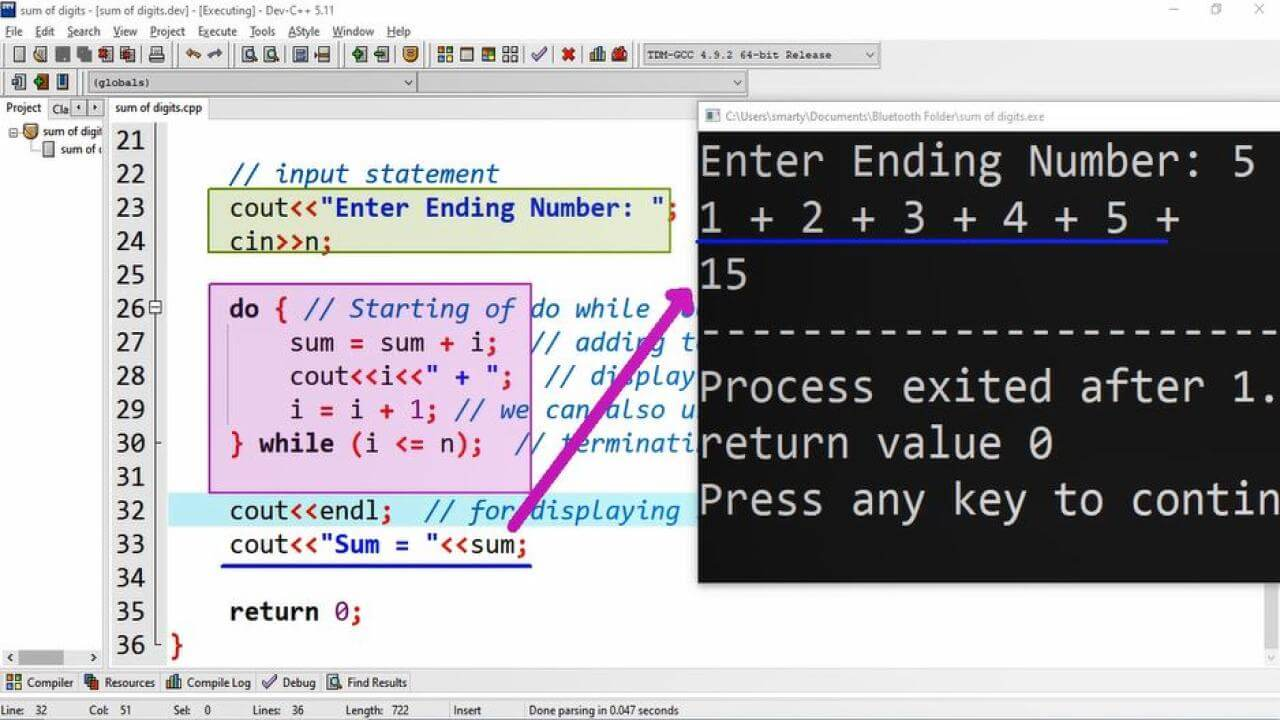# Sum Of Series Using Do While Loop in CPP

## Source Code:

#include <iostream>

// standard namespace
using namespace std;

/*
if series is upto 10
eg:
1,2,3,4,5,6,7,8,9,10
then sum =
1+2+3+4+5+6+7+8+9+10
*/

int main(int argc, char** argv) {

//Declaration statement
int i = 1;
int n; // N value from user
// initialization statement
int sum = 0; // To store sum of numbers

// input statement
cout<<"Enter Ending Number: ";
cin>>n;

do { // Starting of do while loop
sum = sum + i;  // adding to sum variable
cout<<i<<" + ";  // displaying seris in output
i = i + 1; // we can also use i++
} while (i <= n);  // terminating condition

cout<<endl;  // for displaying sum in new line
cout<<"Sum = "<<sum;

return 0;
}

## Output:## Working:

We can calculate sum of natural numbers by adding up all these numbers to sum common storage/variable. This program implement the logic of sum of series using do while loop. For this purpose first of all all we declare the follwing variables.

 Sr. Identifier Data Type Description 1 i int This is used as counter variable. 2 n int n variable will store the nth number of series taken by user input. 3 sum int sum variable is initialized with addition identity 0. It will be used to store sum.

After declaration we use do while loop, in loop body counter variable is incremented and also print numbers using cout statement. This program example is written and compiled using Dv-C++ IDE.